# Life Sciences - (XL) 2018 GATE Paper (Practice Test - 1)

## 25 Questions MCQ Test GATE Past Year Papers for Practice (All Branches) | Life Sciences - (XL) 2018 GATE Paper (Practice Test - 1)

Description
Attempt Life Sciences - (XL) 2018 GATE Paper (Practice Test - 1) | 25 questions in 30 minutes | Mock test for GATE preparation | Free important questions MCQ to study GATE Past Year Papers for Practice (All Branches) for GATE Exam | Download free PDF with solutions
QUESTION: 1

Solution:
QUESTION: 2

Solution:
QUESTION: 3

### Seven machines take 7 minutes to make 7 identical toys. At the same rate, how many minutes would it take for 100 machines to make 100 toys?

Solution:
QUESTION: 4

A rectangle becomes a square when its length and breadth are reduced by 10 m and 5 m, respectively. During this process, the rectangle loses 650 m2 of area. What is the area of the original rectangle in square meters?

Solution:
QUESTION: 5

A number consists of two digits. The sum of the digits is 9. If 45 is subtracted from the number, its digits are interchanged. What is the number?

Solution:
QUESTION: 6

For integers a, b and c, what would be the minimum and maximum values respectively of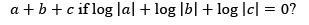Solution:
QUESTION: 7

Given that a and b are integers and a + b2 c3 is odd, which one of the following statements is correct?

Solution:
QUESTION: 8

From the time the front of a train enters a platform, it takes 25 seconds for the back of the train to leave the platform, while travelling at a constant speed of 54 km/h. At the same speed, it takes 14 seconds to pass a man running at 9 km/h in the same direction as the train. What is the length of the train and that of the platform in meters, respectively?

Solution:
QUESTION: 9

Which of the following functions describe the graph shown in the below figure?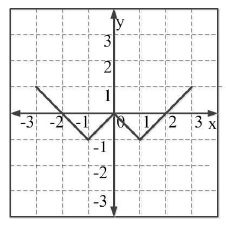Solution:
QUESTION: 10

Consider the following three statements:

(i) Some roses are red.
(ii) All red flowers fade quickly.
Which of the following statements can be logically inferred from the above statements?

Solution:
QUESTION: 11

For the complete combustion of graphite and diamond in oxygen individually, the standard enthalpy change (ΔHo298) values are -393.5 kJ mol-1 and -395.4 kJ mol-1, respectively. Then, the ΔHo298 for the conversion of graphite into diamond is

Solution:
QUESTION: 12

For a 4s orbital of hydrogen atom, the magnetic quantum number (ml) is

Solution:
QUESTION: 13

Hybridization of xenon in XeF2 is

Solution:
QUESTION: 14

Two equivalents of P react with one equivalent of Q to produce a major product R.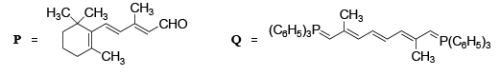The number of double bonds present in the major product R is _______.

Solution:
QUESTION: 15

The total number of possible stereoisomers for the compound with the structural formula CH3CH(OH)CH=CHCH2CH3 is _______.

Solution:
QUESTION: 16

Among B-H, C-H, N-H and Si-H bonds in BH3, CH4, NH3 and SiH4, respectively, the polarity of the bond which is shown INCORRECTLY is

Solution:
QUESTION: 17

Among the following statements,

(i) [NiCl4]2- (atomic number of Ni = 28) is diamagnetic

(ii) Ethylamine is a weaker Lewis base compared to pyridine

(iii) [NiCl2{P(C6H5)3}2] has two geometrical isomers

(iv) Bond angle in H2O is greater than that in H2S,the

CORRECT one is

Solution:
QUESTION: 18

In [Mn(H2O)6]2+ (atomic number of Mn = 25), the d-d transitions according to crystal field theory (CFT) are

Solution:
QUESTION: 19

The major product M in the reaction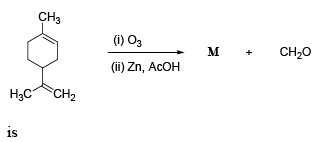Solution:
QUESTION: 20

The two major products of the reaction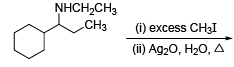are

Solution:
QUESTION: 21

The compound, which upon mono-nitration using a mixture of HNO3 and H2SO4, does NOT give the meta-isomer as the major product, is

Solution:
QUESTION: 22

The standard reduction potential (Eo) for the conversion of Cr2O72- to Cr3+ at 25 °C in an aqueous solution of pH 3.0 is 1.33 V. The concentrations of Cr2O72- and Cr3+ are 1.0 × 10-4 M and 1.0 × 10-3 M, respectively. Then the potential of this half-cell reaction is  (Given: Faraday constant = 96500 C mol-1, Gas constant R = 8.314 J K-1 mol-1)

Solution:
QUESTION: 23

The solubility product (Ksp) of Mg(OH)2 at 25 °C is 5.6 × 10-11. Its solubility in water is . S × 10-2 g/L, where the value of S is _______ (up to two decimal places). (Given: Molecular weight of Mg(OH)2 = 58.3 g mol-1

Solution:
QUESTION: 24

The activation energy (Ea) values for two reactions carried out at 25 °C differ by 5.0 kJ mol-1. If the pre-exponential factors (A1 and A2) for these two reactions are of the same magnitude, the ratio of rate constants (k1/k2) is _______ (up to two decimal places). (Given: Gas constant R = 8.314 J K-1 mol-1)

Solution:
QUESTION: 25

One mole of helium gas in an isolated system undergoes a reversible isothermal expansion at 25 °C from an initial volume of 2.0 liters to a final volume of 10.0 liters. The change in entropy (DS) of the surroundings is ______ J K-1 (up to two decimal places). (Given: Gas constant R = 8.314 J K-1 mol-1)

Solution:Use Code STAYHOME200 and get INR 200 additional OFF Use Coupon Code